﻿ Numerical Analysis of the Hydrodynamic Performance of HATST with Different Blade GeometriesPublications are Open
Access in this journal
Article Versions
Export Article
• Normal Style
• MLA Style
• APA Style
• Chicago Style
Research Article
Open Access Peer-reviewed

### Numerical Analysis of the Hydrodynamic Performance of HATST with Different Blade Geometries

Alireza Abbasi, Hassan Ghassemi , David Molyneux
American Journal of Civil Engineering and Architecture. 2018, 6(6), 236-241. DOI: 10.12691/ajcea-6-6-2
Received October 12, 2018; Revised November 20, 2018; Accepted November 28, 2018

### Abstract

This paper is presented to the hydrodynamic performance of horizontal axis tidal stream turbine (HATST) with different blade geometries. The Reynolds-averaged Navier-Stokes (RANS) equations solver of the Star-CCM+ software is employed with a realizable k-ε turbulent model. The turbine has three blades and different blade NACA sections. The numerical results of pressure distribution, power and thrust coefficients (CP and CT) are presented and discussed at various tip speed ratios (TSR). It is found that the maximum power coefficients are obtained around 0.37 to 0.41 at TSR between 4 and 5. The results of thrust coefficients are found that 6418 is more than other sections.

### 1. Introduction

The ocean waves and currents are an important sources of energy that mankind can use of these resources to meet their needs with the benefit from the knowledge. The uses of renewable energies cause reduce fossil fuel consumption, and they may be used only for specific needs and also can save them. This will protect both the environment against greenhouse gas emissions and industrial sewage from fossil fuels and also more endless energy of nature is to be used. Apart from the seas and oceans, there are other energy sources such as wind and solar but because of the seas and oceans are divided into various energy sources including tidal energy, wave energy, and energy of marine current and the amount of the energy from them is more predictable than other sources, more used from them. Comprehensive study of the all marine renewable energy types described out by Nazari in his master thesis. Then, he designed a point absorber energy for the Persian Gulf 1, 2.

The tidal turbines and wind turbines are almost similar from the geometrically and physically point of view. Therefore, the aerodynamic and hydrodynamic performances are almost the same. There are two types of the tidal turbine like wind turbines, i.e. vertical axis and horizontal axis turbines. The horizontal-axis turbines are more efficient than vertical-axis turbines because these turbines that their function is on a lifting force basis, have caused that the vertical-axis turbines less used.

In recent years, major researches about the tidal energy are dedicated to horizontal-axis turbines because the installation of large-sized vertical-axis turbines are very effortful and its electricity generation capacity is less than that of horizontal-axis turbines.

Computational fluid dynamic (CFD) is a best method for studying complex flows around turbines because it is possible to obtain good and acceptable results by simulating these flows with the help of CFD. One of the disadvantages of numerical methods is high computational costs.

Many researchers carried out on the tidal turbine by numerical methods and experimental measurements. Bhaj et al performed the power and thrust measurements of marine current turbines under various hydrodynamic flow conditions in a cavitation tunnel and a towing tank 3. Hydrodynamic design of HATST have numerically predicted 4, 5. Xu et al. employed numerical techniques for the design and prediction of performance of marine turbines and propellers 6. Shi et al. carried out the hydrodynamic characteristics of a three-bladed tidal turbine with a flow separation effect on the turbine efficiency by ANSYS CFX software 7. Numerical method employed to the analysis of the three-blade tidal turbine using CFD, i.e. presented by Gunawan et al. 8.

Noruzi et al. performed design, analysis and performance of the hydrokinetic of horizontal marine current axial turbine, considering the depth of the turbine installation 9. To model the high amplitude of wave ocean wave, they applied linear wave theory for gravity waves. The actuator disk theory to display the tidal turbine was simulated 10. In their research, several turbulence models used to simulate the wake behind a turbine represented by a porous disk are compared. The tested models were the Standard and the realizable k-ε models, the SST k-ω model and the Reynolds stress model (RSM).

A study on the wake field of tidal current turbines with different numerical methods was conducted by Liu et al. 11. In their investigation the rotational effects of the three-bladed turbine were simulated with both the moving reference frame (MRF) and the sliding meshes techniques. Numerical and experimental investigation on the performance of three newly designed 100 kW-class tidal current turbines carried out by Song et al 12. Recently, experimental study on kinetic energy conversion carried out by Seo et al 13, design and hydrodynamic analysis of HATST with winglets presented by 14 and hydrodynamic performance including of the HATST using RANS solver carried performed by Ghassemi et al 15.

The paper sections are organized as follows. Section 2 describes the HATST geometry and section types. In section 3, governing equations, solution domain, boundary conditions and computational grid are presented. The numerical results are presented and discussed in section 4, and finally conclusions are given in section 5.

### 2. HATST Geometry and Section Types

The geometric characteristics of the HATST are selected in diameter 0.72 [m] and 3-bladeed with different sections types. At each radial section, the chord and twist angle are different. Table 1 is given the chord length and twist angle each radial section from root to tip.

• Figure 1. 3D model of T-4418

The first blade section that used in all sections of HATST blade is NACA4418 the difference is that the twist angle and length of the chord changes in each section. All information about this horizontal axis turbine such as numerical and experimental results is given in 14. In this study, to 3D modeling of HATST and change their geometry in order to generate four other HATSTs, CATIA software was used Figure 1. The 3D models of other HATSTs are shown in Figure 2. Hereafter, instead of the NACA- 4digits, it is named the T-4digits, as an example; NACA4418 is named T-4418.

• Figure 2. 3D model of T-2418, T6418, T-4412 and T-4425

### 3. Computational Method

3.1. Mathematical Formulation

The governing equations of the turbulent incompressible flow include the continuity and Navier-Stokes equations. By applying Reynolds averaging, RANS equations will be obtained: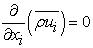(1)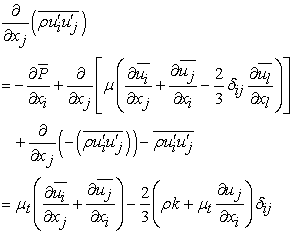(2)

whereindicates Reynolds stresses. Therefore, the Reynolds stresses are given by: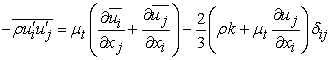(3)

whereis the turbulent viscosity and k is the turbulent kinetic energy.

The most commonly used turbulence models are two-equation models that basis of their function is the creating a relationship between the turbulence viscosity with longitudinal scales and turbulence velocities. In these models, there is a good equilibrium between computational costs and the accuracy of the results and these models are perfect.

All two-equation turbulence models use turbulence kinetic energy (k) as the first variable is given while the second transfer equation is written for unknown variable, the turbulence models differ with each other. One of the standard model and practical in the industry of the turbulence currents in the CFD is the k-ε model and this model has good precision and stability. This turbulence model is divided into three sub-models including Standard k-ε, realisable k-ε, RNG k-ε that the realisable k-ε turbulence model is used in present study. The modeled transport equations for k and ε for steady-state and incompressible flow, are given by:(4)(5)

In Equations (4) and (5),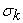andare the turbulent Prandtl numbers for k and ε respectively. The production of turbulence kinetic energy,is approximated in a manner consistent with the Boussinesq hypothesis by:(6)

S is the modulus of the mean rate-of-strain tensor, defined by: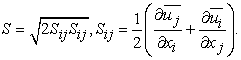(7)

The dissipation of this turbulence kinetic energy,is defined by:(8)

The production and diffusion terms in the transport equation for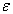differ slightly and are defined as:(9)

whereis a constant, while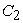is defined as: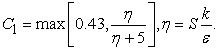(10)

The turbulent viscosity is computed through a formulation of k and, given as: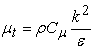(11)is calculated using the following equations: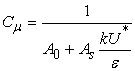(12)

where: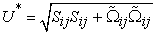(13)(14)is a constant and the remaining variable,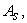is calculated using the following:(15)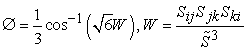(16)

whereand the constants applied in the realisable k-ε turbulence model are equal to:The hydrodynamic characteristics of the HATST may be defined using the power coefficient (CP), thrust coefficient (CT) and tip speed ratio (TSR). These equations are defined as follows: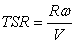(17)(18)(19)

where R is the radius of the blade and V is the flow speed and Q (N.m) is torque and T (N) is thrust and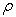is water density (kg/m3).

3.2. Computational Domain and Boundary Conditions

To simulate an engineering problem, firstly a simple geometry should be chosen as a computational domain that in this case, flow equations of fluid are solved by determining boundary conditions and applying forces and information of the problem. Choosing the computational domain and geometry dimensions should be done accurately because otherwise the errors of simulation results will increase and maybe non-physical phenomena will occur. In this study, two cylinders are used to simulate the flow around the turbine and determining the computational domain. Two cylinders are modeled in large and small scales. The small cylinder is designated to simulate the rotational flow around the HATST and its diameter is 1.2D that the D is rotor diameter. Also the large cylinder is designated to simulate the stationary flow around the HATST that its diameter is 5D and its length at the upstream of the HATST and its length in the downstream of the HATST are 3D and 6D, respectively.

The boundary conditions that used in this study include velocity inlet, pressure outlet, non-slip condition and symmetry plane. In inlet field of current at the upstream, the boundary condition of velocity inlet is used and pressure outlet boundary condition is used for outlet field of current at the downstream. For this reason, that the velocity gradient perpendicular to the symmetry plane is zero, boundary condition of symmetry plane is considered for outward surface of stationary region that its distance from the rotational region boundary is 2D approximately. Since the calculation of lift and drag forces on the rotor surface is important, the non-slip boundary condition has been chosen for rotor walls. Boundary conditions and computational domain around the HATST (T-4418) are shown in Figure 3.

• Figure 3. Boundary conditions and computational domain
3.3. Mesh Generation

In the present study, unstructured polyhedral mesh is used for meshing of the computational domain and conformal mesh have been used for interface of between rotational and stationary regions in order to consolidate the mesh and reduce the error of results.

To accurately compute the velocity alteration in the boundary layer around the HATST, the mesh of blades and its surrounding area in the rotational region should be finer than the other parts. Also the grids become larger by approaching the boundaries of the computational region. The existence of sufficient mesh number and its size at the boundary layer is one of the important issues in simulation of turbulent flow, especially when the physical phenomena near the wall are important. Momentum equations are simplifying by using the hypotheses to compute the velocity profile near the wall. Then, the equations are solved with analytical methods. The computed functions in this method are named wall functions. Near the wall can be used the following dimensionless functions: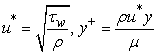(20)

where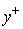is defined for the first node near the wall andis friction velocity. If thevalue is high, the first computational node is placed outside the boundary layer. If theis very fine, the first node is placed below the viscose sub layer that in this case, cannot be used the wall functions. That's why, thevalue should be considered appropriate so in this study, thevalue is 30. In order to independence the results to the mesh and obtain the appropriate accuracy, various five types of mesh sizes have been used to compute the power coefficient (CP) in the TSR 4. Figure 4 shows the mesh generation around the T-4418. Figure 5 presents the effect of mesh size on CP of the T-4418 at TSR=4.

• Figure 4. Mesh generated around the T-4418
• Figure 5. Mesh independency for T-4418 at TSR=4

As can be seen in the Figure 5, no change in the results will be obtained as the number of meshes increases. Therefore, the number of 1625978 meshes has been used to avoid increasing the computational costs and analysis time.

### 4. Results and Discussion

In this part, the results of numerical simulation are compared with experimental data. Comparing the numerical results with experimental data is performed to get the precision and verification of the numerical results. Figure 6 shows the comparison between power coefficient (CP) of the T-4418 and the experimental results at various TSR. The CFD results are in good agreement with experimental data. The maximum value of CP is around 0.4~0.41 at TSR=4~4.5 for both numerical and experimental.

Figure 7 and Figure 8 are presented the power and thrust coefficients for five blade section types. The maximum power coefficient is given at TSR between 4 and 5 while the thrust coefficient is increase when the TSR increases.

• Figure 6. Comparison of power coefficient of T-4418
• Figure 7. Power coefficient for five blade types
• Figure 8. Thrust coefficient for five blade types

Three blades of the T-2412, T-4418 and T-4412 give more power coefficient while two others (T-4425 and T-6418) give lower power especially when the TSR in increased. Maximum power coefficients for all of them are obtained around 0.37 to 0.41 at TSR 4 to 5.

Thrust coefficient is presented in Figure 8 at various TSR from 3 to 7. All of them increase when the TSR increases with different slopes. At TSR<4 the thrust coefficients are increased with steep slope but at TSR>4 increase moderate. For 5 blade sections, the results of thrust coefficients are found that T-2418<T-4425<T-4418<T-4412<T-6418 for all TSR.

### 5. Conclusions

This paper is presented the numerical results of the power coefficients, thrust coefficients and pressure distribution of the different blade section types of the HATST by Star-CCM+ solver. The k-ε turbulence model is used to reach better results. The turbine has 3 blades, diameter is 0.72 [m] and the five types of the blade sections. Based on the simulation and numerical results, it is concluded that the performance of the T-2418 is better and its maximum power coefficient 0.406 at the TSR=4.5, also T-4425 has the lowest performance among other blades types.

In addition, at the low velocities the operation of T-6418 is good but at the high velocities its operation is less than the other turbines. Finally, power coefficient of T-4412 is similar to T-2418 in all TSRs except the TSRs of 3 and 7 have suitable value.

### References

  Nazari, M., Study and design of the point absorber wave energy for Persian Gulf, Master Thesis, Amirkabir University of Tech, 2011. In article  Nazari, M., Ghassemi H., Ghiasi M., Sayehbani M., Design of the point absorber wave energy converter for Assaluyeh Port, Iranica Journal of Energy and Environment, 4 (2), 130-135. In article  Bahaj, A.S., Molland, A.F., Chaplin, J.R., Batten, W.M.J., Power and thrust measurements of marine current turbines under various hydrodynamic flow conditions in a cavitation tunnel and a towing tank. Renew. Energy 32 (3), 2007, 407-426. In article View Article  Batten, W.M.J., Bahaj, A.S., Molland, A.F., Chaplin, J. R,. Experimentally Validated Numerical Method for the Hydrodynamic Design of Horizontal Axis Tidal Turbines. Ocean Eng. 34, 2007. In article View Article  Batten W.M.J., Bahaj A.S., Molland A.F., J.R. Chaplin, The prediction of the hydrodynamic performance of marine current turbines, Renewable Energy, 33, 2008, 1085-1096. In article View Article  Xu W., Numerical Techniques for the Design and Prediction of Performance of Marine Turbines and Propellers, Master of Engineering Thesis in Department of Civil, Architectural and Environmental Eng., The University of Texas at Austin, 2010. In article  Shi W., Wang D., Atlar M., Seo K..,. Flow separation impacts on the hydrodynamic performance analysis of a marine current turbine using CFD, Proc. Inst. Mech. Eng. Part A 227, 2013, 833-846. In article View Article  Gunawan, B.M.C., Model validation using experimental measurements from the Garfield Thomas water tunnel at the applied research laboratory at Penn State University. In: Proceedings of the 2nd Marine Energy Technology Symposium, 2014. In article  Noruzi R., Vahidzadeh M., Riasi A., Design, analysis and predicting hydrokinetic performance of a horizontal marine current axial turbine by consideration of turbine installation depth, Ocean Eng. 108, 2015, 789-798. In article View Article  Nguyen V. T., Guillou S. S., Thiebot J., Cruz A. S., Modeling turbulence with an Actuator Disk representing a tidal turbine, Renewable Energy. 97, 2016, 625-635. In article View Article  Liu J., Lin H., Purimitla S. R., Wake field studies of tidal current turbines with different numerical methods, Ocean Engineering 117, 2016, 383-397. In article View Article  Song, Museok, Kim, Moon-Chan, Do, In-Rok, Hyung Rhee, Shin, Hyun Lee, Ju, Hyun, Beom-Soo, Numerical and experimental investigation on the performance of three newly designed 100 kW-class tidal current turbines. Inter J. Nav. Archit. oc. Eng. 4, 2012, 241-255. In article  Seo, Jeonghwa, Lee, Seung-Jae, Choi, Woo-Sik, Park, Sung Taek, Hyung Rhee, Shin, Experimental study on kinetic energy conversion of horizontal axis tidal turbine. Renew. Energy 97, 2016, 784-797. In article View Article  Ren, Y., Liu, B., Zhang, T., Fang, Q., Design and hydrodynamic analysis of horizontal axis tidal stream turbines with winglets. Ocean. Eng. 144, 2017, 374-383. In article View Article  Ghassemi H, Ghafari H.R., Homayoun E., Hydrodynamic performance of the horizontal axis tidal stream turbine using RANS solver, Scientific Journals of the Maritime University of Szczecin, 2018, 55 (127), 23-33. In article

Published with license by Science and Education Publishing, Copyright © 2018 Alireza Abbasi, Hassan Ghassemi and David MolyneuxThis work is licensed under a Creative Commons Attribution 4.0 International License. To view a copy of this license, visit http://creativecommons.org/licenses/by/4.0/